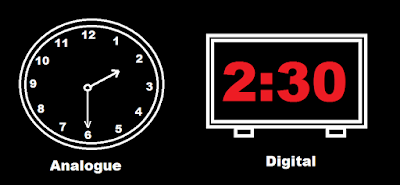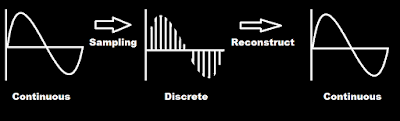# Sampling of Analogue Signals

Sampling of Analogue Signals

Sampling of Analogue Signals•Sampling means splitting in regular interval to convert it in digital form.

•Sampling converts continuous signal to discrete signal.

•Actually the accuracy of analogue signal is more than digital because digital signal have finite data.

•All the positions in discrete signal is a number.

•So analogue signal have some different advantages and digital signal have some different advantages.

•Sampling is used in measurement system and data converters.

•By using different sampling rate we can sampled the signal.

•Sampling frequency or sampling rate Fs = 1/T is the number of samples obtained in one second.

•If the samples are less then it will cause aliasing which will cause loss in information.

•Using Nyquist theorem (Fs ≥2B) where 2B is Nyquist rate we can avoid aliasing and decide how much sampling frequency should use.

•Using sampling we can store the analogue data in digital form.

← Prev Question Next Question →

## Related questions

Last answer: Find the phasors corresponding to the following signals